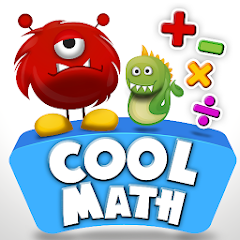# Cool Math Educational Games for 1st 2nd Grade Kids

1K+Everyone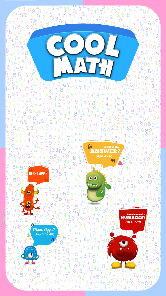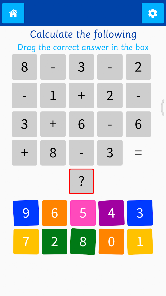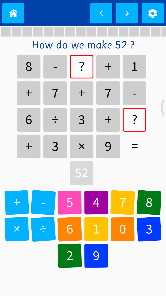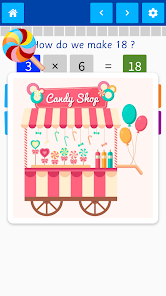Math Education for early grade kids that promotes mental math techniques.

The app is bundled with two math programs

1. Calculate the Following
2. How do we make Numbers

1) “Calculate the Following” generates a very simple question and ask the user to drag the correct answer in the Answer Box. It generates a math question that is primarily built for early learners (grade 1). The question is so simple that the kid doesn’t have to calculate above number 10. The operation is limited to addition and subtraction only.

2) “How do we make Numbers” on the other hand is flexible to be used from 1st grade to any age group. The app generates a math equation where the user has to guess the missing number. Depending on the difficulty level, the operation can involve addition, subtraction, multiplication and division of higher numbers. Also, a question might have two blank pieces to fill.

The app starts with a simple math question and automatically generates difficult questions over progression.

Both programs, in a fun to learn activity, silently develops the following abilities specially in teens and early learners.

• Understanding combination of different numbers produces the same result (2+7 = 5+4).
• Left to right, Top to bottom sequence to solve math equations.
• Builds ability to solve complex problems.
• Understanding about how a set of opposite numbers cancel each other (+5 -5 = +2 – 2).
• Calculated estimation.
• Examination of a product’s construction. (reverse engineering an equation).
• Increases the attention span.
• Mental arithmetic.
• Speed calculations.

The app has several salient features.
• The app is providing many controls to adjust the generated question to suite various requirements.
• The app difficulty level can be made fixed.
• Coins, awards and achievements builds motivation to keep practicing.
• Drag and drop scenario are meditating for kids and they keep their interest in practicing math.
• Randomized, simple yet challenging questions to solve.
• The app’s interface is user friendly and attractive.

NOTE:
1. The app uses left to right sequence for calculation and doesn’t use DMAS rule for few reasons. However we are working to provide it as an additional difficulty level in future versions.

2. The app is provided as a limited version for demonstration and it contains ads, the complete app can be unlocked by using In App purchases within the app. It is strongly recommended that the kids practice the ads-free complete app.

A reset button is provided so the kids can use the app at initial levels.

We look forward for your input to make the app even better. Please consider to support us by rating the app and writing a small review. It also helps us to produce new and better apps.
Updated on
Dec 16, 2020

## Data safety